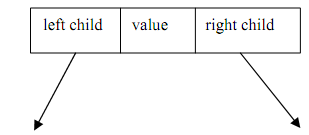## Implementation of a binary tree, Data Structure & Algorithms

Assignment Help:

Like general tree, binary trees are implemented through linked lists. A typical node in a Binary tree has a structure as follows

struct NODE

{

struct NODE *leftchild;

int nodevalue;               /* this can be of any data type */

struct NODE *rightchild;

};Figure: Node structure of a binary tree

The 'left child 'and 'right child' are pointers to another tree-node. The "leaf node" (not shown) here will have NULL values for these pointers.

The binary tree creation follows a extremely simple principle. For the new element to be inserted, compare it along with the current element in the tree. If its value is less than the present element in the tree, then move to the left side of that element or else to its right. If there is no sub tree at the left, then make your new element as the left child of that present element or else compare it to the existing left child and follow the similar rule. Exactly, the same need to done for the case while your new element is greater than the current element into the tree however this time with the right child. Though this logic is followed for the formation of a Binary tree, this logic is frequently suitable to search for a key value in the binary tree.

Following is algorithm for the implementation of Binary tree:

Step-1: If new element < current element, then go to step-2 or else go to step -3

Step-2: If the current element does not contain a left sub-tree, then converts new element into the left child of the current element; else make the existing left child as your current element and go to step-1

Step-3: If the current element does not contain a right sub-tree, then converts new element into the right child of the present element; else make the existing right child as your existing element and go to step-1

#### Infix expression to postfix form using the stack function, Q. Convert the f...

Q. Convert the following given Infix expression to Postfix form using the stack function: x + y * z + ( p * q + r ) * s , Follow general precedence rule and suppose tha

#### Determine the greatest common divisor, Determine the greatest common diviso...

Determine the greatest common divisor (GCD) of two integers, m & n. The algorithm for GCD might be defined as follows: While m is greater than zero: If n is greater than m, s

Double Linked List In a doubly linked list, also known as 2 way lists, each node is separated into 3 parts. The first part is called last pointer field. It has the address of t

#### System defined data types, System defined data types:- These are data t...

System defined data types:- These are data types that have been defined by the compiler of any program. The C language contains 4 basic data types:- Int, float,  char and doubl

#### Postfix expression, : Write an algorithm to evaluate a postfix expression. ...

: Write an algorithm to evaluate a postfix expression. Execute your algorithm using the following postfix expression as your input: a b + c d +*f ­ .

#### Array, how to define the size of array

how to define the size of array

#### Explain multiplication method, Multiplication Method: The multiplication m...

Multiplication Method: The multiplication method operates in 2 steps. In the 1ststep the key value K is multiplied by a constant A in the range O

#### Mid square method, Mid Square method with good example

Mid Square method with good example

#### Hash table, Programming for hash table?

Programming for hash table?

#### Define chaining process of hashing, Chaining In this method, instead of...

Chaining In this method, instead of hashing function value as location we use it as an index into an array of pointers. Every pointer access a chain that holds the element havi